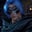Related Tags

python
communitycreator
for-in loop
list comprehension

# How to get Python maximum value keys in the dictionaryGutha Vamsi Krishna

In this shot, we will learn how to get the maximum value keys in the dictionary.

We will start by defining the problem statement, followed by an example.

### Problem statement

Given a dictionary, find all the keys with the maximum value in the dictionary.

### Example

Let’s assume a dictionary with the student name as key and age as value.

Input: {"john":12,"steve":20,"robert":19, "diana":20,"riya":15}

Output: [steve,diana]

Explanation: Only Steve and Diana are 20, which is the maximum value in the given dictionary.

### Implementation

To solve this problem, we will use the following approach:

• Get all values from a dictionary.
• Find out the maximum value.
• Traverse through the dictionary using the for-in loop and list comprehension.
• Check if the present key value equals the maximum value. If it does, then add it to the list.
• Finally, print the list.
#declare dictionary students
students = {
"john":12,"steve":20,"robert":19, "diana":20,"riya":15
}

#find maximum value in dictionary
maximum_value = max(students.values())

#get keys with maximum value using list comprehension
maximum_keys = [key for key in students if students[key]==maximum_value]

#print the maximum keys
print(maximum_keys)

### Explanation

• Line 2: Declare and initialize the dictionary, students.
• Line 7: Get the maximum value in the dictionary using the max() function and pass all dictionary values as a parameter.
• Line 10: Traverse through the dictionary, students, and check if the present key's value is equal to the maximum value. If it is equal, add it to a list using list comprehension.
• Line 13: Print the list of keys that have a maximum value in the dictionary.

RELATED TAGS

python
communitycreator
for-in loop
list comprehension

CONTRIBUTORGutha Vamsi Krishna
RELATED COURSES

View all Courses

Keep Exploring

Learn in-demand tech skills in half the time